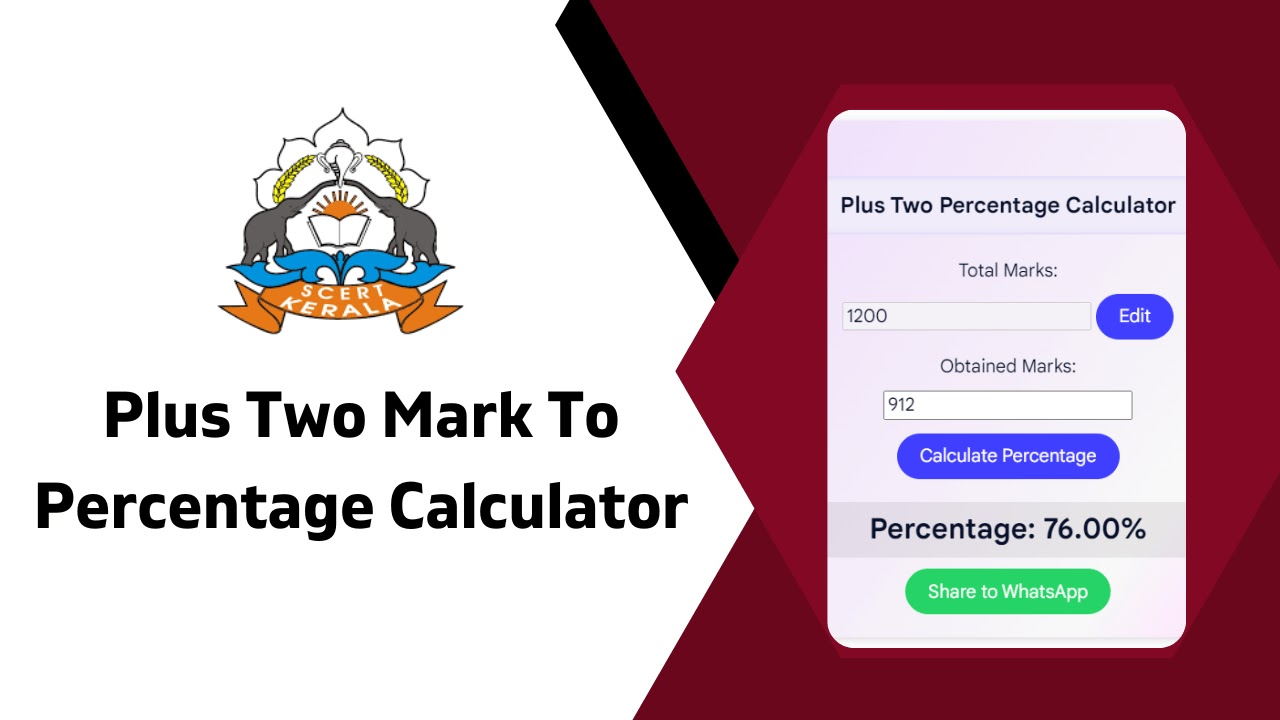നമസ്കാരം,

# Plus Two Mark To Percentage Calculator Online

Plus Two Mark To Percentage Calculator Online:Calculate Kerala Plus Two percentage using our valuable tool. Determine marks to percentage conversion

## Plus Two Mark To Percentage Calculator Online

The Kerala Plus Two Percentage Calculator is a valuable tool for 12th-grade students in Kerala. It enables students to calculate their percentage based on the marks obtained in the final board exams conducted by SCERT, following the NCERT syllabus. Once the Kerala Plus Two results are announced, students can utilize our Plus Two Science, Commerce, Humanities, and other streams' percentage calculator to determine their overall percentage. This calculator helps students calculate their +2 percentage by inputting the marks they have achieved in their exams. With the help of our Kerala Plus Two Percentage Calculator, students can easily evaluate their performance and gain a clear understanding of their academic achievements in the 12th grade.Our Plus Two Percentage Calculator provides insights into various calculations, such as Plus Two percentage calculation, Plus Two mark to percentage conversion, 12th percentage calculation from grade points, as well as how to calculate the percentage of marks in the 10th class. It also assists in determining the 10th mark percentage, understanding how to calculate the 12th percentage, and computing the total marks. By offering a comprehensive range of functionalities, our calculator simplifies the process of calculating percentages and helps students assess their academic progress effectively. Whether in the Science, Commerce, or Humanities streams, our Kerala Plus Two Percentage Calculator empowers students to track their performance accurately and make informed decisions regarding their future educational endeavors.

## Kerala Plus Two Percentage Calculator Online

### Plus Two Percentage Calculator

Copying and using this code on other websites without permission is strictly prohibited. Legal action may be taken against unauthorized use.

### How To Calculate Plus Two Mark To Percentage?

below we give an example :

• Marks Obtained in Subject 1 - 176
• Marks Obtained in Subject 2 - 164
• Marks Obtained in Subject 3 - 171
• Marks Obtained in Subject 4 - 185
• Marks Obtained in Subject 5 - 179
• Marks Obtained in Subject 6 - 187

Total Percentage = ((176+164+171+185+179+187)/1200) X 100

(1062/1200) X 100

The percentage obtained: 88.5 %

In conclusion, the Kerala Plus Two Percentage Calculator serves as a valuable resource for 12th-grade students in Kerala, allowing them to determine their overall percentage based on their exam marks. By inputting their scores into the calculator, students can easily calculate their +2 percentage and gain a comprehensive understanding of their academic performance. Additionally, the calculator offers various functionalities, including mark to percentage conversion, grade point calculation, and assessing the percentage of marks in the 10th class. With its user-friendly interface and accurate calculations, the Kerala Plus Two Percentage Calculator provides students with a convenient and reliable tool to assess their achievements and make informed decisions about their future educational endeavors.

തൊഴിൽ വാർത്തകൾ ഏറ്റവും വേഗത്തിൽ അറിയുവാൻ ജോയിൻ ചെയ്യൂ